# Logarithms: Equations, Systems and Properties

• Introduction

• Remember that... definition of logarithm and its properties

• Resolved Logarithmic Equations, Systems and Demonstration of the properties

## Introduction

A logarithmic equation is an equation that has an unknown factor in the argument of a logarithm. In reality, the resolution is reduced to the resolution of equations of the same type as the expressions in the arguments (quadratic equations, cubic equations, irrational equations...).

This section is a collection of resolved exercises of equations and equation systems of this kind, which are intended to be in order of increasing difficulty.

In the majority of logarithms the base is not specified, because we suppose it's 10. Although, in this sense, we must decide that in the majority of scientific texts, if it does not say otherwise, that the base is e (as it's known, Napierian logarithm).

Also, at the end we will prove the logarithmic properties: logarithm of a product, of a quotient, of a power and base switch.

Regarding the use of the logarithms, we can talk of their frequent use in physics. They appear, for example, to calculate the apparent magnitude of a celestial body (measured by the amount of light we perceive); the magnitude of an earthquake in the Richter scale; the age (time gone by) in radiometric dating (the Carbon 14 test); the pH.

## Remember that...

Before starting the exercises, let's remember the definition of logarithm:

$$log_b (a) = c \Leftrightarrow b^c = a$$

• a is called the base of the logarithm

• b is called the argument of the logarithm

• c the number that

$$b^c = a$$

• So, c is the exponent to which the base b must be raised to be the number a.

$$b^{log_b (c)} = c$$

• If b is the number e, we write ln(x) instead loge (x). This is the Naperian logarithm.

### Logarithm Properties

 logarithm of a product: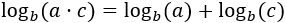logarithm of a quotient:logarithm of a power: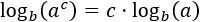change of base: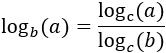Useful property: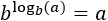# Resolved Logarithmic Equations

Exercise 1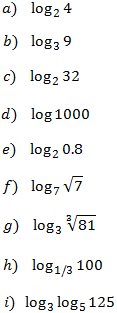Show solution

Equation 1Show solution

Equation 2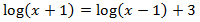Show solution

Equation 3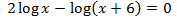Show solution

Equation 4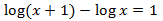Show solution

Equation 5Show solution

Equation 6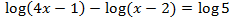Show solution

Equation 7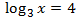Show solution

Equation 8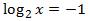Show solution

Equation 9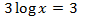Show solution

Equation 10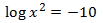Show solution

Equation 11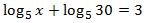Show solution

Equation 12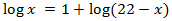Show solution

Equation 13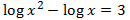Show solution

Equation 14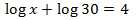Show solution

Equation 15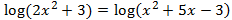Show solution

Equation 16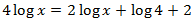Show solution

Equation 17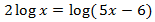Show solution

Equation 18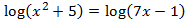Show solution

Equation 19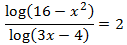Show solution

Equation 20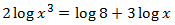Show solution

Equation 21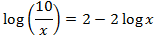Show solution

Equation 22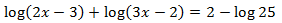Show solution

Equation 23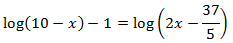Show solution

Equation 24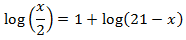Show solution

Equation 25Show solution

# 11 Resolved Systems

System 1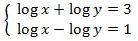Show solution

System 2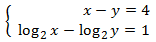Show solution

System 3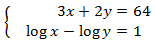Show solution

System 4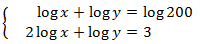Show solution

System 5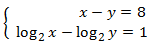Show solution

System 6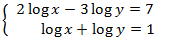Show solution

System 7Show solution

System 8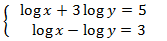Show solution

System 9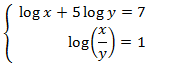Show solution

System 10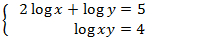Show solution

System 11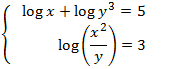Show solution

# Proof of the Properties

Property 1: logarithm of a productShow Proof

Property 2: logarithm of a quotient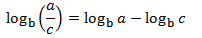Show Proof

Property 3: logarithm of a powerShow Proof

Property 4: change of base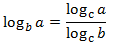Show Proof

Property 5: change of base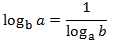Show Proof